Courses

# Application of the First Law to Open Systems Civil Engineering (CE) Notes | EduRev

## Civil Engineering (CE) : Application of the First Law to Open Systems Civil Engineering (CE) Notes | EduRev

The document Application of the First Law to Open Systems Civil Engineering (CE) Notes | EduRev is a part of the Civil Engineering (CE) Course Thermodynamics.
All you need of Civil Engineering (CE) at this link: Civil Engineering (CE)

Application of the First Law to Open Systems

While the last section addressed processes occurring in closed systems, the wider application of the first law involves formulating the energy balance differently in order to accommodate the fact that most thermodynamic systems, i.e., equipments, in continuous process plants are essentially open systems: they allow mass transfer across their boundaries (i.e., through inlet and outlet).  Examples include pumps, compressors, reactors, distillation columns, heat exchangers etc. Since such open systems admit both material and energy transfer across their boundaries the thermodynamic analysis necessarily involves both mass and energy balances to be carried out together. Also such systems may in general operate under both steady (during normal plant operation) and unsteady states (say during startup and shutdown). As we will see the former state is a limiting case of the more general situation of unsteady state behavior.

Mass Balance for Open Systems: For generality consider an open system with which has multiple inlets (1, 2) and outlets (3, 4). The volume enclosed by the physical boundary is the control volume (CV). The general mass balance equation for such a system may be written as: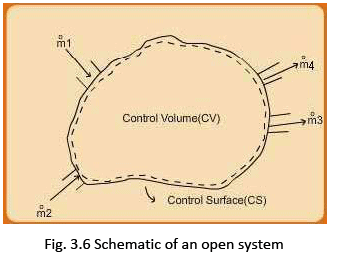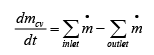...(3.27)

The mass flow rate  m is given by: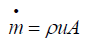.......(3.28)
Where, ρ = fluid density; u = fluid velocity; and A = aperture cross-sectional area The first term on the left side of the eqn. (3.27) denotes sum of all flow rates over all inlets, while the second term corresponds to the summation over all outlet flow rates. For the system shown in fig. 3.5 the eqn. 3.27 may be written as: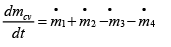...(3.28)

The last equation may be reframed in a general way as follows: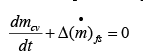...(3.29)

Where the symbol ∆ ≡ oulet-inlet; and the subscript fs stands for "flow streams".
Equation 3.29 may be recast as: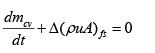...(3.30)

The above equation simplifies under steady flow conditions as the accumulation term for the control volume, i.e.,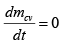In such a case for the simplest case of a system with one inlet and outlet (say: 1 and 2 respectively), which typically represents the majority of process plant equipments, the mass balance equation reduces to:

m =constant

Or: m ρ1 uA1 = ρuA2 → uA1 = uA2 / V2                   ......(3.31)

Where V = specific volume

Energy Balance for Open Systems: Consider the schematic of an open system as shown in fig. 3.7. For simplicity we assume one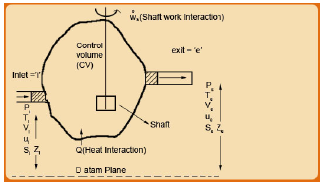Fig. 3.7 Schematic of an open system showing flow and energy interactions

inlet and one exit ports to the control volume. The thermodynamic states at the inlet i and exit e are defined by the P, V, T, u (average fluid velocity across the cross section of the port), and Z, the height of the port above a datum plane. A fluid element (consider an unit mole or mass) enters the CV carrying internal energy, kinetic and potential energies at the inlet conditions (Pi, Ti, with molar volume as Vi) and leaves values of these energies at the exit state conditions (Pe, Te, with molar volume as Ve
Thus the total specific energy of the fluid at the two ports corresponds to the sum of specific internal, potential and kinetic energies, given by: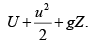In addition, the CV exchanges heat with the surroundings at the rate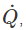, and say a total work (in one or more
forms) at the rate of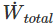In the schematic we, however, have shown a specific work form, shaft work, that is delivered to or by the system by means of rotatory motion of a paddle wheel which, as we will see later in the section, is implicated in many typical process plant units. As with material balance one may write a total energy balance equation for the control volume as follows: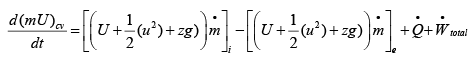or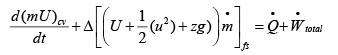..(3.32)

The general total work term should include all forms of work. We draw the reader’s attention to the fact that the total work interaction also should include that needed to push fluid into the CV as well as that implicated in pushing it out of CV.  The fluid state at the inlet or exit is characterized by a set of state properties, U, V, H, etc. Consider a unit mass (or mole) of fluid entering the CV. This fluid element obviously needs to be “pushed” by another that follows it so as to make the formed enter the CV. In essence a fluid element of (specific) volume V is pushed into the CV at a pressure P. This is akin to a P-V form of work (as in the case of a piston-in-a-cylinder system) that is done on the CV and so may be quantified as – Pi Vi . The same considerations apply at the exit in which case in pushing out a similar fluid element at exit conditions, i.e., – PV( ) 1 ( 2 ) )
eqn. 3.32 may be rewritten as follows: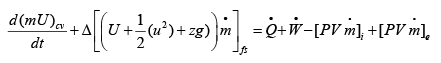...(3.33)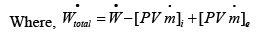Where,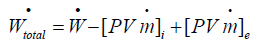The term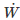represents sum of all other forms of work associated with the process occurring within the CV. This residual work term may include the shaft work, P-V work resulting from expansion or contraction of the CV, electrical work, and so on. As the last two work terms on the left side of the eqn. 3.33 are associated with the flow streams we may rewrite the equation as follows: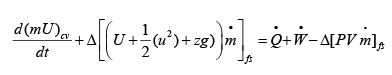...(3.34)

On rearranging: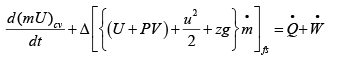...(3.35)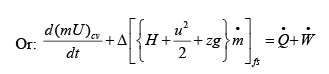...(3.36)

It may be noted that eqn. 3.34 assumes that the CV is fixed in space and therefore no overall potential of kinetic energy terms depicting these mechanical energies for the control volume is included. This, of course, is valid for all process plant applications.  In addition, For many cases of practical importance (though not all) in a chemical plant the kinetic and potential energy changes between the inlet and exit streams may not be significant, whence the last equation may be simplified as: an equipment may be neglected; hence: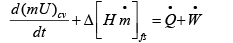...(3.37)

Further, for the special case where the only shaft work is involved, the above equation may be simplified to: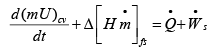...(3.38)

For steady state applications the eqn. 3.34 reduces to:...(3.39)
Further, if the kinetic and potential energy changes associated with the flow streams are insignificant, it follows that: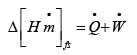...(3.40

Since under steady state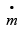is constant we may write:

ΔH = Q + W                    ...(3.41)

Where Q and W denote the work and heat interactions per unit mass of mole of fluid flowing through the system flowing through system. Once again if W s is the only form of work interaction between the system and the surrounding then:

ΔH = Q + Ws                    ...(3.42)

Examples of process plant units to which eqn. 3.42 applies are: pumps, compressors, turbines, fans, blowers, etc. In all cases a rotatory part is used exchange work between the system and surrounding.

Offer running on EduRev: Apply code STAYHOME200 to get INR 200 off on our premium plan EduRev Infinity!

## Thermodynamics

56 videos|92 docs|33 tests

,

,

,

,

,

,

,

,

,

,

,

,

,

,

,

,

,

,

,

,

,

;